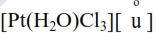Deepak Scored 45->99%ile with Bounce Back Crack Course. You can do it too!

# The reaction rate for the reaction

Question:

The reaction rate for the reaction

$\left[\mathrm{PtCl}_{4}\right]^{2-}+\mathrm{H}_{2} \mathrm{O} \rightleftharpoons\left[\mathrm{Pt}\left(\mathrm{H}_{2} \mathrm{O}\right) \mathrm{Cl}_{3}\right]^{-}+\mathrm{Cl}^{-}$

was measured as a function of concentrations of different species. It was observed that $\frac{-\mathrm{d}\left[\left[\mathrm{PtCl}_{4}\right]^{2-}\right]}{\mathrm{dt}}=4.8 \times 10^{-5}\left[\left[\mathrm{PtCl}_{4}\right]^{2-}\right]-2.4 \times$

$10^{-3}\left[\left[\mathrm{Pt}\left(\mathrm{H}_{2} \mathrm{O}\right) \mathrm{Cl}_{3}\right]^{-}\right]\left[\mathrm{Cl}^{-}\right]$

where square brackets are used to denote molar concentrations. The equilibrium constant $\mathrm{K}_{\mathrm{c}}=$ - (Nearest integer)

Solution:

$\left[\mathrm{PtCl}_{4}\right]^{-2}+\mathrm{H}_{2} \mathrm{O} \rightleftharpoons\left[\mathrm{Pt}\left(\mathrm{H}_{2} \mathrm{O}\right) \mathrm{Cl}_{3}\right]^{-}+\mathrm{Cl}^{-}$

$\frac{-\mathrm{d}\left[\mathrm{Pt} \mathrm{Cl}_{4}\right]^{-2}}{\mathrm{dt}}=4.8 \times 10^{-5}\left[\mathrm{PtCl}_{4}^{-2}\right]-2.4 \times 10^{3}$$\Rightarrow \mathrm{K}_{\mathrm{eq}}=\frac{\mathrm{k}_{\mathrm{f}}}{\mathrm{k}_{\mathrm{b}}}=\frac{4.8 \times 10^{-5}}{2.4 \times 10^{-3}}=0.02$